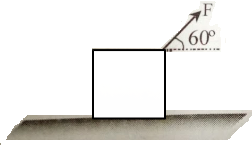A particle is moving in a circle of radius r centered at O with constant speed v. The change in velocity moving from A to B $\left(\angle \mathrm{AOB}=40°\right)$ is -

(A)

(B)

(C)

(D)

Concept Questions :-

Resultant of Vectors
High Yielding Test Series + Question Bank - NEET 2020

Difficulty Level:

If $\stackrel{\to }{A}=\stackrel{\to }{B}+\stackrel{\to }{C}$ and magnitudes of A, B and C are 5, 4 and 3 units respectively, the angle between A and C is -

(A)

(B)

(C)

(D)  $\mathrm{\pi }/2$

Concept Questions :-

Resultant of Vectors
High Yielding Test Series + Question Bank - NEET 2020

Difficulty Level:

What is the resultant of three coplanar forces:  at $0°$ at $30°$ and  at $150°$?

1.

2.

3.

4.

Concept Questions :-

Resultant of Vectors
High Yielding Test Series + Question Bank - NEET 2020

Difficulty Level:

Two forces, ${\mathrm{F}}_{1}$ and ${\mathrm{F}}_{2}$ are acting on a body. One force is doubled of the other force and the resultant is equal to the greater force. Then the angle between the two forces is -

(A)

(B)

(C)

(D)

Concept Questions :-

Resultant of Vectors
High Yielding Test Series + Question Bank - NEET 2020

Difficulty Level:

If the angle between vector a and b is an acute angle, then the difference a$-$b is -

(A)  the main diagonal of the parallelogram

(B)  the minor diagonal of the parallelogram

(C)  any of the above

(D)  none of the above

Concept Questions :-

Resultant of Vectors
High Yielding Test Series + Question Bank - NEET 2020

Difficulty Level:

What displacement must be added to the displacement   to give a displacement of  pointing in the x-direction?

(A)

(B)

(C)

(D)

Concept Questions :-

Resultant of Vectors
High Yielding Test Series + Question Bank - NEET 2020

Difficulty Level:

The value of unit vector in the direction of vector , is -

(A)  $\stackrel{^}{\mathrm{i}}$

(B)  $\stackrel{^}{\mathrm{j}}$

(C)  $\left(\stackrel{^}{\mathrm{i}}+\stackrel{^}{\mathrm{j}}\right)/13$

(D)

Concept Questions :-

Resultant of Vectors

Difficulty Level:

A child pulls a box with a force of  at an angle of $60°$above the horizontal. Then the horizontal and vertical components of the force are -(A)  100 N, 175 N

(B)  86.6 N, 100 N

(C)  100 N, 86.6 N

(D)  100 N, 0 N

Concept Questions :-

Resolution of vectors

Difficulty Level:

A man rows a boat with a speed of  in northwest direction. The shoreline makes an angle of $15°$ south of west. Obtain the component of the velocity of the boat along the shoreline.

(A)

(B)

(C)

(D)

Concept Questions :-

Resolution of vectors
High Yielding Test Series + Question Bank - NEET 2020

Difficulty Level:

A bird moves from point (1, -2) to (4, 2). If the speed of the bird is 10 m/sec, then the velocity vector of the bird is:

(A)  $5\left(\stackrel{^}{\mathrm{i}}-2\stackrel{^}{\mathrm{j}}\right)$

(B)  $5\left(4\stackrel{^}{\mathrm{i}}+2\stackrel{^}{\mathrm{j}}\right)$

(C)  $0.6\stackrel{^}{\mathrm{i}}+0.8\stackrel{^}{\mathrm{j}}$

(D)  $6\stackrel{^}{\mathrm{i}}+8\stackrel{^}{\mathrm{j}}$

Concept Questions :-

Resultant of Vectors